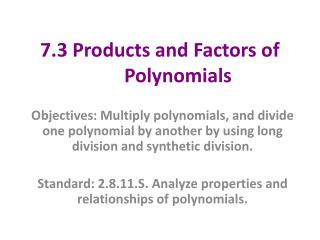DownloadDownload Presentation7.3 Products and Factors of Polynomials

# 7.3 Products and Factors of Polynomials

Download Presentation## 7.3 Products and Factors of Polynomials

- - - - - - - - - - - - - - - - - - - - - - - - - - - E N D - - - - - - - - - - - - - - - - - - - - - - - - - - -
##### Presentation Transcript

1. 7.3 Products and Factors of Polynomials Objectives: Multiply polynomials, and divide one polynomial by another by using long division and synthetic division. Standard: 2.8.11.S. Analyze properties and relationships of polynomials.

2. Example 1. Making an open-top box out of a single rectangular sheet involves cutting and folding square flaps at each of the corners. These flaps are then pasted to the adjacent side to provide reinforcement for the corners. The dimensions of the rectangular sheet and the square flaps determine the volume of the resulting box. For the 12-inch-by-16-inch sheet shown, the volume function is V(x) = x(16 – 2x)(12 – 2x), where x is the side length in inches of the square flap. Write the volume function for the open-top box as a polynomial function in standard form. V(x) = x(16 – 2x)(12 – 2x) (16x – 2x2) (12 – 2x) 192x – 32x2 – 24x2 + 4x3 4x3 – 56x2 + 192x

3. Ex 2A. Write f(x) = 2x2 (x2 + 2) (x - 3) as a polynomial in standard form. (2x4 + 4x2) (x – 3) 2x5 – 6x4 + 4x3 – 12x2 Ex. 2B Write as a polynomial in standard form.

4. Just as a quadratic function is factored by writing it as the product of 2 factors, a Polynomial Expression is in FACTORED FORM when it is written as the product of 2 or more factors.

5. Ex 3. Factor each polynomial. • x3 - 5x2 - 6x • x3 + 4x2+2x + 8 • x3– 9x x (x2 – 9) x (x + 3) (x – 3) • x3– x2+ 2x – 2 x2 (x – 1) + 2 (x – 1) (x2 + 2) ( x – 1)

6. c. x3 + 1000 d. x3 – 125 (x + 10) (x2 – 10x + 100) (x2 + 5x + 25) (x – 5)

7. Factor Theorem x – r is a factor of the polynomial expression that defines the function P if and only if r is a solution of P(x) = 0, that is, if and only if P(r) = 0. With the Factor Theorem, you can test for linear factors involving integers by using substitution.

8. Use substitution to determine whether x + 3 is a factor of x3 – 3x2 – 6x + 8. • Solution is x = - 3. • (-3)3 – 3(-3)2 – 6(-3) + 8 • -27 – 27 + 18 + 8 • -28 • No, its not a factor.

9. DIVIDING POLYNOMIALS BY SYNTHETIC OR LONG DIVISION A polynomial can be divided by a divisor of the form x – r (FIRST POWER) by using long division or a shortened form of long division called synthetic division.

10. You can only use Synthetic Division if you’re dividing by a LINEAR factor (x+ a)

11. * Find the quotient.(x3 + 3x2 – 13x – 15) ÷ (x2 – 2x – 3) The quotient is: x + 5

12. Given that -3 is a zero of P(x) = x3 - 13x – 12, use synthetic division to factor x3 - 13x – 12. The Quotient is x2 - 3x - 4 x2 - 3x – 4 (x - 4)(x + 1)(x + 3) (zeros are x = 4, x = -1, and x = -3)

13. Remainder Theorem If the polynomial expression that defines the function of P is divided by x – a, then the remainder

14. Ex 12. Given P(x) = 3x3 - 4x2 + 9x + 5, find P(6) by using both synthetic division and substitution. P(6) = 563